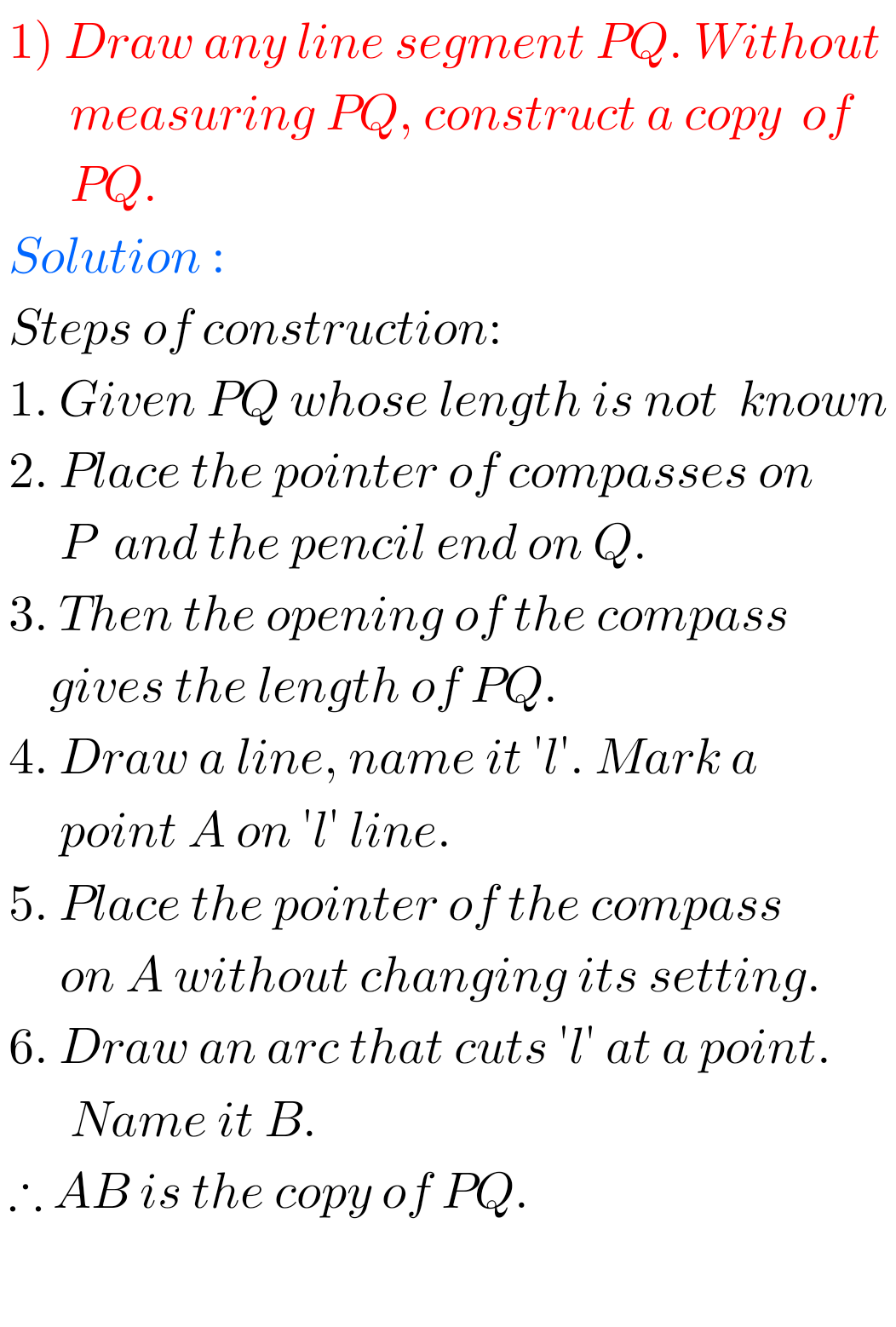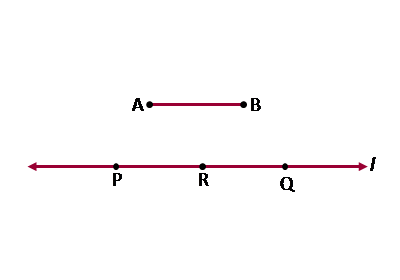# Practical Geometry Solutions Class 6 Ncert Exercise 14.3

Ncert solutions for Mathematics Class 6th text book Chapter 14 Practical Geometry Exercise 14.3 are given.

Study the textbook lesson Practical Geometry very well.

Observe the example problem and solutions given in the textbook.

Observe the given below solutions and try them in your own method.

You can see the solutions for Ncert Maths Class 6 Practical Geometry

Chapter 1 Knowing our Numbers

Chapter 2 whole Numbers

Chapter 3 Playing with Numbers

Chapter 6 Integers

Chapter 14 Practical Geometry

Exercise 14.1

Exercise 14.2

M

# Chapter 14 Practical Geometry Solutions Class 6 Ncert exercise 14.3

Chapter 14 Practical Geometry Exercise 14.3 Ncert Maths

Exercise 14.3## Ncert Solutions for class 6 Practical Geometry exercise 14.3Note : Observe the solutions and try them in your own method.